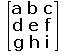# the affine and projective groups

We have observed that, regarded as groups of transformations of R2,
we have the inclusions E(2) ≤ S(2) ≤ A(2). Also, S(2) is isomorphic to
the subgroup S(2) = {t ε I(2) : t(∞) = ∞} of I(2).

In a similar way, we show that A(2) is isomorphic to a geometrically
defined subgroup of P(2). The key is the observation that an affine
transformation maps the plan to itself, and maps families of parallel
lines to families of parallel lines. Thus, we can think of an affine
transformation as mapping ideal points to ideal points.

Suppose that t is the projective transformation with the matrix A
given on the right. Then t maps the plane Π : z=0 to itself if and
only if, for all x,y, t maps [x,y,0] to a p-point with the third
coordinate equal to zero i.e. gx + hy + i0 = 0. Since this holds
for all x,y, we must have g = h = 0. As A is non-singular, i ≠ 0.
Finally, since t may also be given by λA, we may assume i = 1.

Then a simple calculation shows that A maps (x,y,1) to the point
(ax+by+c,dx+ey+f,1). This maps Π' : z = 1 to itself, and acts on Π'
like the affine transformation of the plane mapping the point (x,y)
to (ax+by+c,dx+ey+f).

Conversely, an affine transformation of the above form leads to a
projective transformation with matrix having rows (a b c), (d e f)
and (0 0 1).

Definition
The subgroup A(2) of P(2) consists of all elements with matrix
A = (aij) having a31 = a32 = 0.

The above calculation shows that A(2) is isomorphic to A(2).

We can interpret our proof as choosing a p-line L in RP2, which
plays the role of the "ideal line". We consider the subgroup H
of P(2) consisting of the elements which fix L. Although the
algebra is more involved, we can show that H acts like the affine
group on any plane parallel to the "plane" corresponding to L.

The relation between the groups leads to a relation between
their fundamental theorems.

 A  =